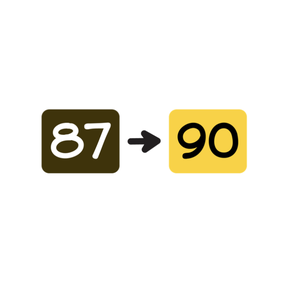Rounding to the nearest ten- numbers to 100

# Rounding to the nearest ten- numbers to 100

Rounding to the nearest ten- numbers to 100.

No account needed.8,000 schools use Gynzy92,000 teachers use Gynzy1,600,000 students use Gynzy

## General

Students learn to round numbers to 100 to their nearest ten. They know when to round up and when to round down.

## Relevance

It is important to be able to round so you can calculate faster. You can use this to estimate totals and make calculations easier. An example is if you want to buy a TV that costs \$98, you know that you need about \$100 dollars.

## Introduction

Students practice setting numbers to 100 on the number line. They determine which numbers are being indicated. Emphasize the nearest ten to the number. Do this by counting from the nearest ten.

## Development

Discuss what tens are and explain that they are good landmark numbers. Tens end on a zero. Ask students which numbers they can name that are not tens. Explain that rounding is turning a random number into a tens number. And the number is rounded up or down based on its nearest ten. Using a number line show the nearest ten first with a number rounding up, then with a number rounding down.
Next show students that they can use the ones place to determine the nearest ten. If the number is 4 or less, you round down. If the number is 5 or higher, you round up. Have students practice with a TO(tens-ones) chart and then again without the TO chart.

Check that students understand how to round to the nearest ten by asking the following questions:
- When do you round up?
- When do you round down?
- Do you look at the first number or the last number to determine if you round up or down? Why?
- Which tens does 85 round to?

## Guided practice

Students first round up or down using the number line. Next they choose between rounding options and finally they determine the nearest ten on their own.

## Closing

Discuss with students that it is important to be able to round numbers because you can estimate amounts that way. Check with a closing exercise that students are able to round to the nearest ten of a given number. Show students a selection of numbers one by one. If the number indicates that they should round up, they should stand up. If the number indicates that they should round down, they should sit down. Ask students why they round a number up or down. Emphasize that when rounding to tens that they have to look at the last number.

## Teaching tips

For students who have difficulty rounding, have them use a TO chart, or use the number line to help visualize the process.

### The online teaching platform for interactive whiteboards and displays in schools

• Save time building lessons

• Manage the classroom more efficiently

• Increase student engagement